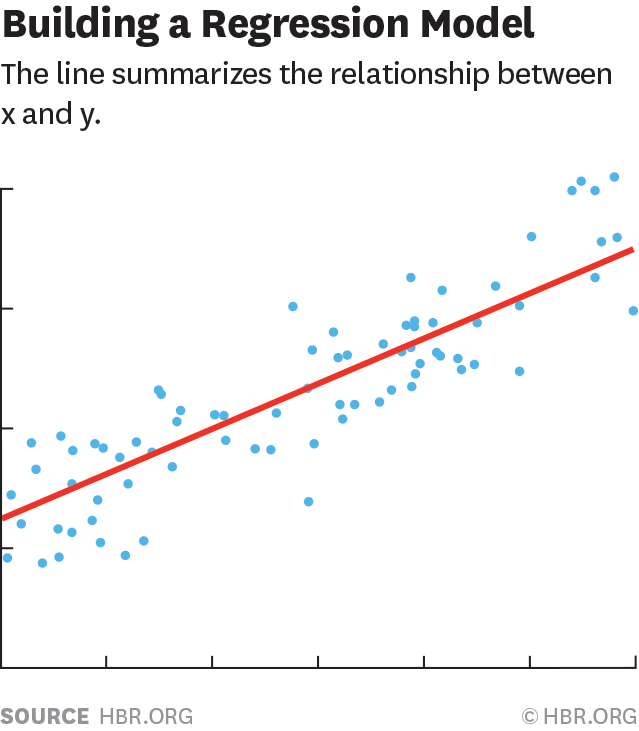# Simple Linear regression model

Source: Deep Learning on Medium# Simple linear regression model

artificial intelligence has been an ever growing land field in the world as well as in Africa. The particular case of linear regression is the “hello world” of the domain, a simple based algorithm which can solve many encountered problems such as:

• Predict whether a tumor is malignant or benign, in real-estate domain, weather predictions, and so on..lets dive into the issue :)

firstly we need to know what is regression. regression is simply modeling an output(target) in function of independent inputs called predictors. In the case of simple linear regression, we only have one independent variable (x) and a dependent variable (y).

we only talk of linear regression when we have a straight line which best fit the distribution of our data as shown below

The general equation of a simple linear model is:

Y=aX + b

The objective is to find the values of a and b which best fits our data , these variables are some hyperparameters of our model. before diving into the algorithm, lets look at some basic concepts:

## Lost function:

Our linear model predict a target known as y_pred the lost function is the square difference between the predicted value, y_predict and the real value y_truth of a training example , mathematically we have: